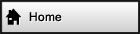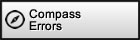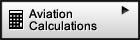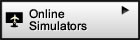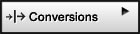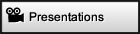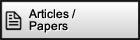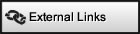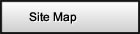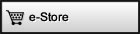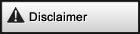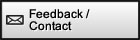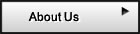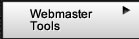Surf with confidence!   Site has been tested by:Norton™ Safe WebMcAfee SiteAdvisor ®Altimetry More precise than your average e6b: Highlight field, type in the unit and get the conversion for all other unitsTemperature ConversionCloud BaseAltitude Correction for Pressure AltitudeTrue AltitudePressure AltitudeTrue AirspeedQNH (altimeter setting)Temperature RiseQFE (zero height setting)Mach NumberDensity AltitudeEquivalent AirspeedTemperature Conversion
 Temperature [oF] [oC] [oR] [K]

Enter a number in any box and get all the remaining box's resultsAltitude Correction for Pressure Altitude
 Altimeter Setting [inHg] [HPa] or [mbar] Altitude Correction [ft] [m]

Enter the altimeter setting or altitude correctionPressure Altitude
 Calibrated Altitude [ft] [m] Altimeter Setting (QNH) [inHg] [HPa] or [mbar] Pressure Altitude [ft] [m]
or
 Absolute Atmospheric Pressue [inHg] [HPa] or [mbar] Pressure Altitude [ft] [m]

If using the top portion (1st calculator), enter any two fields (i.e. Altitude, Altimeter Setting or Pressure Altitude) then
press "Eval" on any remaining field for that field's result. If using the lower portion (2nd calculator), enter the Absolute
Atmospheric Pressure or Pressure Altitude and see the remaining field be calculated.

QNH is a term not commonly used in the United States but used in many other countries. It is the altimeter setting setting
such that the altimeter reads altitude above mean sea level at the airport level. In the U.S. this is referred to as the altimeter
setting and is usually given by ATC (Air Traffic Control) or the airport ATIS (Airport Terminal Information Service)
among other sources.

Note 1: It does not matter the order that you enter the rows. The remaining row (field) will be
calculated based on the values of the other two rows when the Eval button of that row is pressed.

Note 2: If you don't have Calibrated Altitude (CA) use Indicated Altitude.

Note 3: Absolute Atmospheric Pressure is also refered to as Absolute Barometric Pressure.QNH (Altimeter Setting)
 Airport Altitude [ft] [m] Pressure Altitude at Airport [ft] [m] Altimeter Setting (QNH) [inHg] [HPa] or [mbar]

Enter any two fields (i.e. Altitude, Altimeter Setting or Pressure Altitude)
then press "Eval" on any remaining field for that field's result

QNH is a term not commonly used in the United States but used in many other countries. It is the altimeter setting
such that the altimeter reads altitude above mean sea level at the airport level. In the U.S. this is referred to as the altimeter
setting and is usually given by ATC (Air Traffic Control) or the airport ATIS (Airport Terminal Information Service) among
other sources.
Pressure Altitude is the altitude the altimeter reads when set to the QNE setting 1013.25 hPa or
approximately 29.92 inHg. QNE is also a term used more internationally but denotes the altimeter setting to obtain the

Note that it does not matter the order that you enter the rows. The remaining row (field) will be
calculated based on the values of the other two rows when the Eval button of that row is pressed.QFE
 Pressure Altitude at Airport [ft] [m] QFE Setting [inHg] [HPa] or [mbar]

Enter the pressure altitude at airport or the QFE setting
QFE is a term also not commonly used in the United States but referred to in many other countries. In practice it is not used
very much but it is useful to know of it's existence. It is the altimeter setting such that the altimeter reads zero height at the
airport level. Pressure altitude is the altitude the altimeter reads when set to the QNE setting 1013.25 hPa or approximately
29.92 inHg. QNE is also a term used more internationally but denotes the altimeter setting to obtain the pressure altitudeDensity Altitude
 Pressure Altitude [ft] [m] Temperature [oF] [oC] Density Altitude [ft] [m]

Enter any two fields (i.e. Pressure Altitude, Temperature or Density Altitude) then press "Eval" on any remaining field
for that field's result

Note that it does not matter the order that you enter the rows. The remaining row (field) will be
calculated based on the values of the other two rows when the Eval button of that row is pressed.

RELATED WEBSITES:
Wahiduddin's Website (Interesting website with a very thorough explanation of density altitude)
Link to a very complete density altitude calculator and discussion of the effects of humidityCloud Base
Main Variables
 Air Temperature at Ground Level [oF] [oC] Dewpoint at Ground Level [oF] [oC] Cloud Base Altitude Above Ground Level (AGL) [ft] [m]

Correlated Variables
 Temperature/Dewpoint Spread [oF] [oC] Estimated Freezing Level Above Ground [ft] [m] Estimated Temperature at Cloud Base [oF] [oC]
 Enter any two fields (i.e. Air Temperature at Ground Level, Dewpoint at Ground Level or Cloud Base Altitude Above Ground Level) then press "Eval" on any remaining field for that field's result. Other useful information that can be derived will also be displayed on the "Correlated Variables" section. Note that it does not matter the order that you enter the rows. The remaining row (field) will be calculated based on the values of the other two rows when the Eval button of that row is pressed.True Altitude
 Pressure Altitude (PA) [ft] [m] True Air Temperature (T) [oF] [oC] Calibrated Altitude (CA) [ft] [m] Station Altitude [ft] [m] True Altitude (TA) [ft] [m] or Pressure Altitude (PA) [ft] [m] True Air Temperature (T) [oF] [oC] Altimeter Setting (QNH) [inHg] [HPa] or [mbar] Station Altitude [ft] [m] True Altitude (TA) [ft] [m] or Calibrated Altitude (CA) [ft] [m] True Air Temperature (T) [oF] [oC] Altimeter Setting (QNH) [inHg] [HPa] or [mbar] Station Altitude [ft] [m] True Altitude (TA) [ft] [m]

Enter any four fields then press "Eval" on any remaining field for that field's result. True Altitude computations are
inherently imprecise, please be cautious, particularly when extrapolating beyond typical conditions.     Note 1: If you don't have the Station Altitude set it to zero

Note 2: If you don't have Calibrated Altitude (CA) use Indicated Altitude

Note 3: It does not matter the order that you enter the rows. The remaining row (field) will be
calculated based on the values of the other rows when the Eval button of that row is pressed.

Note 4: True Air Temperature (T) is also referred to as Outside Air Temperature (OAT) or Static Air Temperature (SAT).
True Air Temperature (T) is sometimes abbreviated (TAT). It is NOT to be confused to Total Air Temperature also
abbreviated (TAT) which is the same as Indicated Temperature (IAT) or Ram Air Temperatures (RAT).True
A
irspeed

 Correct for compressibility (more precise at high airspeeds) and supersonic speeds. Note: Most E6B flight computers do not adequately factor compressibility at high airspeeds and supersonic flight regime. Therefore if you are trying to compare the results from this calculator with an E6B and most flight computers you should uncheck this box.
Main Variables
Pressure Altitude (PA)     [ft]   [m]
Recovery Coefficient (CT)
Indicated Air Temperature (IAT)     [oF]   [oC]
Calibrated Airspeed (CAS)     [knots]   [Km/h]
True Airspeed (TAS)     [knots]   [Km/h]

Correlated Variables
 True Air Temperature (T) [oF] [oC] Mach (M) Pitot Tube Impact Pressure (qc) [inHg] [HPa] or [mbar] Static Pressure (P) [inHg] [HPa] or [mbar] Pitot Tube Total Pressure (Pt=qc+P) [inHg] [HPa] or [mbar] Speed of Sound (CS) [knots] [Km/h] Temperature Rise (ΔT=IAT-T) [ΔoF] [ΔoC]
or
Main Variables
 Pressure Altitude (PA) [ft] [m] Calibrated Airspeed (CAS) [knots] [Km/h] True Air Temperature (T) [oF] [oC] True Airspeed (TAS) [knots] [Km/h]

Correlated Variables
 Mach (M) Pitot Tube Impact Pressure (qc) [inHg] [HPa] or [mbar] Static Pressure (P) [inHg] [HPa] or [mbar] Pitot Tube Total Pressure (Pt=qc+P) [inHg] [HPa] or [mbar] Speed of Sound (CS) [knots] [Km/h] Indicated Air Temperature (IAT) if CT=1.0 [oF] [oC]

Enter any four fields in white on the upper portion or three fields in white on the lower portion then press "Eval" on
that portion for any remaining field for that field's result. True Airspeed computations are somewhat imprecise, please be
cautious, particularly when extrapolating beyond typical conditions. When calculating the Recovery Coefficient there is a
vary narrow range of solutions. The model used takes into consideration compressibility and may be used for speeds
beyond Mach 1. Correlated Variables display other information and are calculated from results once Eval has been pressed.

Note 1: If you don't know the recovery coefficient set it to 1.0.

Note 2: If you don't have the Calibrated Airspeed (CAS) use Indicated Airespeed (IAS) in place of CAS.

Note 3: True Air Temperature (T) is also referred to as Outside Air Temperature (OAT) or Static Air Temperature (SAT).
True Air Temperature (T) is sometimes abbreviated TAT. It is NOT to be confused to Total Air Temperature also
abbreviated TAT which is the same as Indicated Temperature (IAT) or Ram Air Temperatures (RAT).

Note 4: Indicated Temperature (IAT) is also referd to as Total Air Temperature (TAT) or Ram Air Temperature (RAT).

Total Air Temperature (TAT) is NOT to be confused to True Air Temperature also abbreviated TAT (see note 2 above)

Note 5: It does not matter the order that you enter the rows. The remaining row (field) will be
calculated based on the values of the other rows when the Eval button of that row is pressed.Temperature Rise
 True Airspeed (TAS) [knots] [Km/h] Recovery Coefficient (CT) Temperature Rise (ΔT=IAT-T) [ΔoF] [ΔoC]

Enter any two fields then press "Eval" on any remaining field for that field's result. When calculating the Recovery Coefficient
there is a vary narrow range of solutions. The model used takes into consideration compressibility and may be used for speeds
beyond Mach 1. To calculate True Air Temperature (T) use T=
IAT-ΔT. Where IAT is the Indicated Air Temperature and ΔT is
the Temperature Rise.

Note 1: If you don't know the Recovery Coefficient (CT) set it to 1.0.

Note 2: True Air Temperature (T) is also referred to as Outside Air Temperature (OAT) or Static Air Temperature (SAT).
True Air Temperature (T) is sometimes abbreviated TAT. It is NOT to be confused to Total Air Temperature also
abbreviated TAT which is the same as Indicated Temperature (IAT) or Ram Air Temperatures (RAT).

Note 3: Indicated Air Temperature (IAT) is also referd to as Total Air Temperature (TAT) or Ram Air Temperature (RAT).

Total Air Temperature (TAT) is NOT to be confused to True Air Temperature also abbreviated TAT (see note 2 above)

Note 4: It does not matter the order that you enter the rows. The remaining row (field) will be
calculated based on the values of the other rows when the Eval button of that row is pressed.Mach
Number

 Correct for compressibility (more precise at high airspeeds) and supersonic speeds. Note: Most E6B flight computers do not adequately factor compressibility at high airspeeds and supersonic flight regime. Therefore if you are trying to compare the results from this calculator with an E6B and most flight computers you should uncheck this box.
Main Variables
 Pressure Altitude (PA) [ft] [m] Calibrated Airspeed (CAS) [knots] [Km/h] Mach Number (M)

Correlated Variables
 Pitot Tube Impact Pressure (qc) [inHg] [HPa] or [mbar] Static Pressure (P) [inHg] [HPa] or [mbar] Pitot Tube Total Pressure (Pt=qc+P) [inHg] [HPa] or [mbar]
or
Main Variables
 True Airspeed (TAS) [knots] [Km/h] Recovery Coefficient (CT) Indicated Air Temperature (IAT) [oF] [oC] Mach Number (M)

Correlated Variables
 True Air Temperature (T) [oF] [oC] Speed of Sound (CS) [knots] [Km/h] Temperature Rise (ΔT=IAT-T) [ΔoF] [ΔoC]
or
Main Variables
 True Airspeed (TAS) [knots] [Km/h] True Air Temperature (T) [oF] [oC] Mach Number (M)

Correlated Variables
 Speed of Sound (CS) [knots] [Km/h] Indicated Air Temperature (IAT) if CT=1.0 [oF] [oC]

Enter any two fields in white on the upper portion, three in the middle, or two fields on the lower portion then press "Eval"
on that portion for any remaining field for that field's result. Other useful information that can be derived will also be displayed
on the "Correlated Variables" section.

Note 1: If you don't know the recovery coefficient set it to 1.0.

Note 2: Indicated Air Temperature (IAT) is also referd to as Total Air Temperature (TAT) or Ram Air Temperature (RAT).
Total Air Temperature (TAT) is NOT to be confused to True Air Temperature also abbreviated TAT. True Air
Temperature (TAT) or (T) is also referred to as Outside Air Temperature (OAT) or Static Air Temperature (SAT)

Note 3: It does not matter the order that you enter the rows. The remaining row (field) will be
calculated based on the values of the other rows when the Eval button of that row is pressed.Equivalent
Airspeed

 Correct for compressibility (more precise at high airspeeds) and supersonic speeds. Note: Most E6B flight computers do not adequately factor compressibility at high airspeeds and supersonic flight regime. Therefore if you are trying to compare the results from this calculator with an E6B and most flight computers you should uncheck this box.
Main Variables
 Pressure Altitude (PA) [ft] [m] True Air Temperature (T) [oF] [oC] True Airspeed (TAS) [knots] [Km/h] Equivalent Airspeed (EAS) [knots] [Km/h]

Correlated Variables
 Calibrated Airspeed (CAS) [knots] [Km/h] Mach (M) Pitot Tube Impact Pressure (qc) [inHg] [HPa] or [mbar] Static Pressure (P) [inHg] [HPa] or [mbar] Pitot Tube Total Pressure (Pt=qc+P) [inHg] [HPa] or [mbar] Speed of Sound (CS) [knots] [Km/h]

Enter any three fields in white then press "Eval" on any remaining field for that field's result. Other useful information that
can be derived will also be displayed on the "Correlated Variables" section.

Note 1: True Air Temperature (T) is also referred to as Outside Air Temperature (OAT) or Static Air Temperature (SAT).
True Air Temperature (T) is sometimes abbreviated TAT. It is NOT to be confused to Total Air Temperature also
abbreviated TAT which is the same as Indicated Temperature (IAT) or Ram Air Temperatures (RAT).

Note 2: It does not matter the order that you enter the rows. The remaining row (field) will be
calculated based on the values of the other rows when the Eval button of that row is pressed.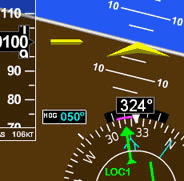Navigation Simulator Practice in our simulator specifically designed for learning the basics of instrument navigation. Try the Navigation Simulator, available for Windows. Free to try fully functional version for 10 days, \$39.95 to buy now free. Note: this simulator has its own self-contained Flash so it works without Flash being installed and is not subject to the Adobe Flash discontnuation / end of life.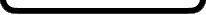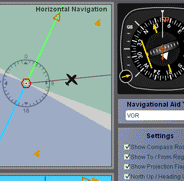Free Online Simulators No installation required. These simulators are ready to run on your web browser and have a rich set of features. Practice basic VOR, ADF, RMI and HSI intrument orientation and execute holding patterns. Other simulators include pitot static system and altimeter errors. Click here to go to main online simulator page.All rights reserved to Luiz Roberto Monteiro de Oliveira.  No content or code of this web site may be reproduced without prior permission from the author.